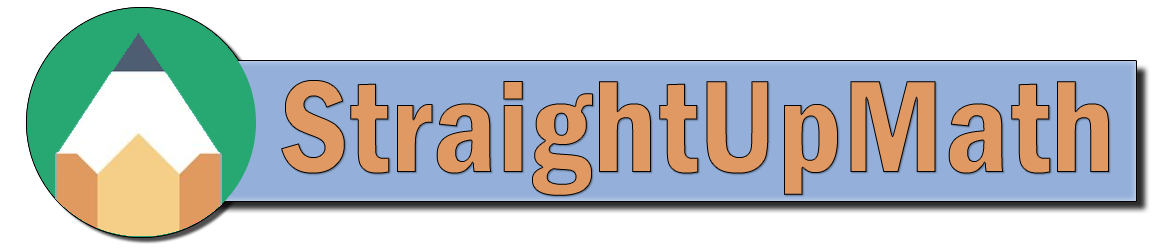Basics - Multiplying

Sorry, no video for this problem at this time.

## Practice

Find the product.
a)    4 × 8 =
32

b)    5 × 9 =
45

c)    7 × 7 =
49

d)    4 × 4 =
16

e)    6 × 4 =
24

f)    6 × 4 =
24

g)    9 × 0 =
0

h)    7 × 3 =
21

i)    6 × 9 =
54

j)    3 × 3 =
9